# Print Longest Common Subsequence | (DP – 26)

Problem Statement:

Print Longest Common Subsequence

In the previous article Longest Common Subsequence, we learned to print the length of the longest common subsequence of two strings. In this article, we will learn to print the actual string of the longest common subsequence.

Prereq: Longest Common Subsequence

Intuition:

Let us consider the following example:We will continue from where we left in the article DP-25. There in the tabulation approach, we declared a dp array and dp[n][m] will have the length of the longest common subsequence., i.e dp[n][m] = 3.

Now, with help of two nested loops, if we print the dp array, it will look like this: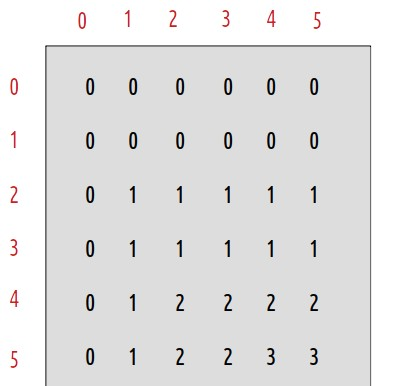Here dp gives us the length of the longest common subsequence: 3.

Now let us try to form the string itself. We know its length already. We give it the name str.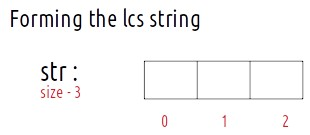We will use the dp array to form the LCS string. For that, we need to think, about how did the dp array was originally filled. The tabulation approach used 1-based indexing. We also write the characters corresponding to the indexes of the dp array: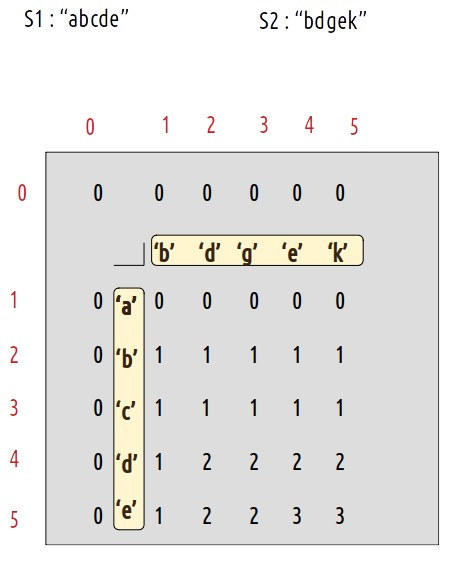Now, let us see what were the conditions that we used while forming the dp array:

• if(S1[i-1] == S2[j-1]), then return 1 + dp[i-1][j-1]
• if(S1[i-1] != S2[j-1]) , then return 0 + max(dp[i-1][j],dp[i][j-1])

These two conditions along with the dp array give us all the required information required to print the LCS string.

### Approach:

The algorithm approach is stated below:

• We will fill the string str from the last by maintaining a pointer.
• We will start from the right-most cell of the dp array, initially i=n and j=m.
• At every cell, we will check if S1[i-1] == S2[j-1], if it is then it means this character is a part of the longest common substring. So we will push it to the str (at last). Then we will move to the diagonally top-left(↖)  cell by assigning i to i-1 and j to j-1.
• Else, this character is not a part of the longest common subsequence. It means that originally this cell got its value from its left cell (←) or from its top cell (↑). Whichever cell’s value will be more of the two, we will move to that cell.
• We will continue till i>0 and j>0, failing it we will break from the loop.
• At last we will get our lcs string in “str”.

### Dry Run:At starting i=5 and j=5.

• As S1 != S2, we move to the left side (←) as it’s value is greater than the top value(↑), therefore i=5 and j=4
• As S1 == S2, we add the current character to the str string(at last) and move to i-1 and j-1 cell i.e top-left(↖), therefore i=4 and j=3.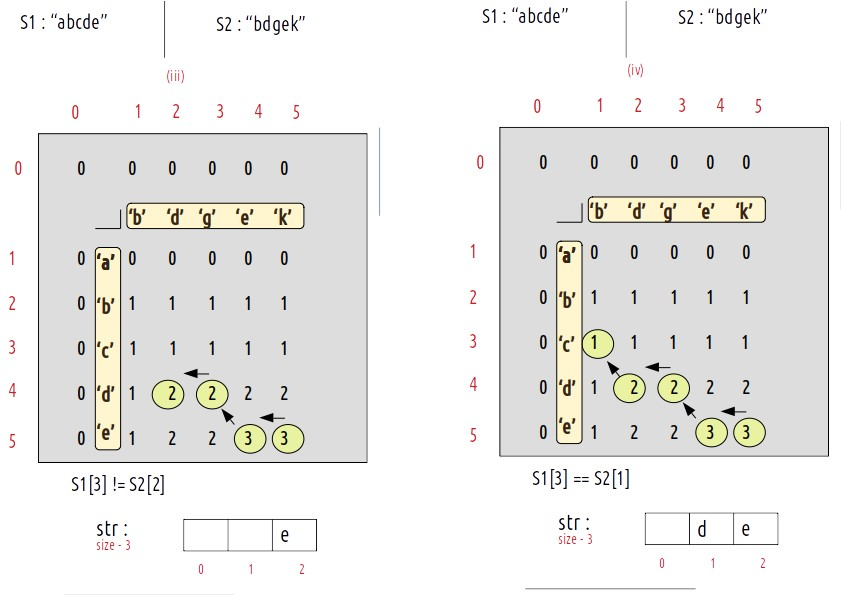• As S1 != S2, we move to the left cell (←) as its value is larger than the top cell(↑), i becomes 4 and j becomes 2.
• As S1 == S2, we will add this character to str string and we will move to the top-left cell (↖) i becomes 3 and j becomes 1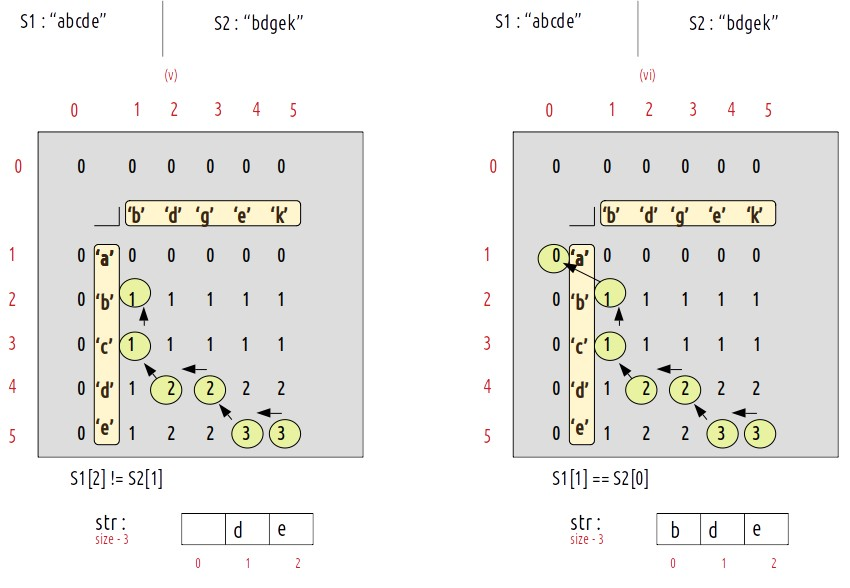• As S1 != S2, we will move to the top cell (↑) as its value is greater than the left cell (←). Now i become 2 and j remains 1.
• As S1 == S2, we will add this character to str string and we will move to the top-left cell (↖) i becomes 1 and j becomes 1 and j becomes 0.
• As j is zero, we have hit the exit condition so we will break out of the loop and str contains the longest common subsequence.

Code:

## C++ Code

``````#include <bits/stdc++.h>

using namespace std;

void lcs(string s1, string s2) {

int n = s1.size();
int m = s2.size();

vector < vector < int >> dp(n + 1, vector < int > (m + 1, 0));
for (int i = 0; i <= n; i++) {
dp[i] = 0;
}
for (int i = 0; i <= m; i++) {
dp[i] = 0;
}

for (int ind1 = 1; ind1 <= n; ind1++) {
for (int ind2 = 1; ind2 <= m; ind2++) {
if (s1[ind1 - 1] == s2[ind2 - 1])
dp[ind1][ind2] = 1 + dp[ind1 - 1][ind2 - 1];
else
dp[ind1][ind2] = 0 + max(dp[ind1 - 1][ind2], dp[ind1][ind2 - 1]);
}
}

int len = dp[n][m];
int i = n;
int j = m;

int index = len - 1;
string str = "";
for (int k = 1; k <= len; k++) {
str += "\$"; // dummy string
}

while (i > 0 && j > 0) {
if (s1[i - 1] == s2[j - 1]) {
str[index] = s1[i - 1];
index--;
i--;
j--;
} else if (s1[i - 1] > s2[j - 1]) {
i--;
} else j--;
}
cout << str;
}

int main() {

string s1 = "abcde";
string s2 = "bdgek";

cout << "The Longest Common Subsequence is ";
lcs(s1, s2);
}``````

Output: The Longest Common Subsequence is bde

Time Complexity: O(N*M)

Reason: There are two nested loops

Space Complexity: O(N*M)

Reason: We are using an external array of size ‘N*M’. Stack Space is eliminated.

## Java Code

``````import java.util.*;

class TUF{
static void lcs(String s1, String s2) {

int n=s1.length();
int m=s2.length();

int dp[][]=new int[n+1][m+1];
for(int i=0;i<=n;i++){
dp[i] = 0;
}
for(int i=0;i<=m;i++){
dp[i] = 0;
}

for(int ind1=1;ind1<=n;ind1++){
for(int ind2=1;ind2<=m;ind2++){
if(s1.charAt(ind1-1)==s2.charAt(ind2-1))
dp[ind1][ind2] = 1 + dp[ind1-1][ind2-1];
else
dp[ind1][ind2] = 0 + Math.max(dp[ind1-1][ind2],dp[ind1][ind2-1]);
}
}

int len=dp[n][m];
int i=n;
int j=m;

int index = len-1;
String str="";
for(int k=1; k<=len;k++){
str +="\$"; // dummy string
}
StringBuilder ss= new StringBuilder(s1);
StringBuilder str2=new StringBuilder(str);
while(i>0 && j>0){
if(ss.charAt(i-1) == s2.charAt(j-1)){
str2.setCharAt(index,ss.charAt(i-1) );
index--;
i--;
j--;
}
else if(ss.charAt(i-1)>s2.charAt(j-1)){
i--;
}
else j--;
}
System.out.println(str2);
}

public static void main(String args[]) {

String s1= "abcde";
String s2= "bdgek";

System.out.print("The Longest Common Subsequence is ");
lcs(s1,s2);
}
}``````

Output: The Longest Common Subsequence is bde

Time Complexity: O(N*M)

Reason: There are two nested loops

Space Complexity: O(N*M)

Reason: We are using an external array of size ‘N*M’. Stack Space is eliminated.

Special thanks to Anshuman Sharma for contributing to this article on takeUforward. If you also wish to share your knowledge with the takeUforward fam, please check out this article